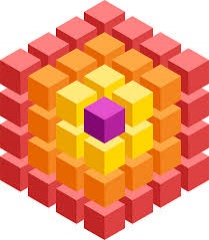Calculate

Calculate the surface of the cube if its volume is 64 cm ^ 3.

S =  96 cm2

Step-by-step explanation:Did you find an error or inaccuracy? Feel free to write us. Thank you!Tips to related online calculators
Tip: Our volume units converter will help you with the conversion of volume units.

You need to know the following knowledge to solve this word math problem:

We encourage you to watch this tutorial video on this math problem: